# Gentle Introduction to CNN

So you’ve been hearing a lot about Convolutional Neural Networks (CNN) but you don’t know what it is, how it works or why it’s all the fuzz now. Let me explain the best way I can!

# Convolution

First and foremost we must understand what a convolution, the building block of CNNs, is.

Let’s say we have a grayscale image, where each pixel has the value of the intensity.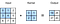From: Convolutions for images

So what are we doing here? We are taking a 3x3 image which is represented as the leftmost matrix, then we have what we call a filter, which is the matrix named “Kernel” (these names kernel and filter are both used to reference the same thing, I will use the name filter for the rest of this post), the output is obtained by multiplying each value of the image with the overlapping value in the filter which is 0x0 + 1x1 + 2x3 + 4x3 = 1 + 6 + 12 = 19, which is what we have in the output in blue. So we take the top left value in the filter and put it in the top left value in the input, we multiply it, so we do 0x0. We take the top right value of the filter and we multiply it by the top right value in the input, we do 1x1. And so on.

## What are the filters

So you have just learned that we do a convolution of an image with a filter but what are filters and what can they do?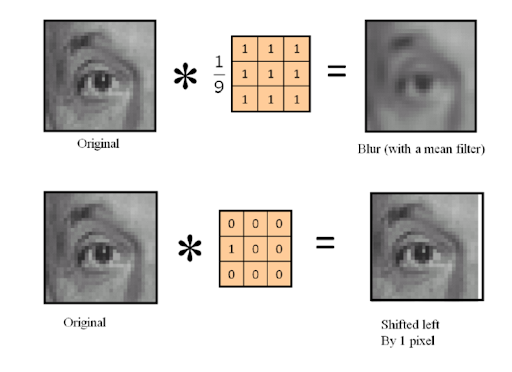From: Image Kernels and Convolutions

We can do various operations like apply a blur, shift the image and much more, I’ll give some more examples but it’s important to notice that we use the same 3x3 filter for the whole image, this is because these features can be repeated for whole image, we don’t need filters that are very big. Let’s look at this example, we want to classify an image as a geometric figure, so we need to detect its edges. We could for instance do something like this: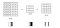From: Edge Detection

which would get the vertical edges (from left to right), you can see in the output we get the edge of 30 in the middle. This is because an edge happens when there’s a big change in the image, like passing from 10 to 0, a big change means a big gradient so that’s what we approximate (not very well) with this filter, the gradient. Then we get the horizontal edges using the other filter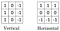From: More On Edge Detection

Or we could also use a filter for edge detection which is called the Sobel filter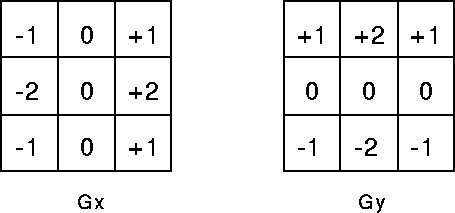From: Wikipedia

From which we can do the convolution of each of these filters with the image (calling the outputs Ox and Oy), where the filter Gy approximates the vertical derivative and the filter Gx approximates the horizontal derivative, then take the outputs Ox and Oy and do

which will approximate the combined gradient amplitude and use this to generate the corners like this: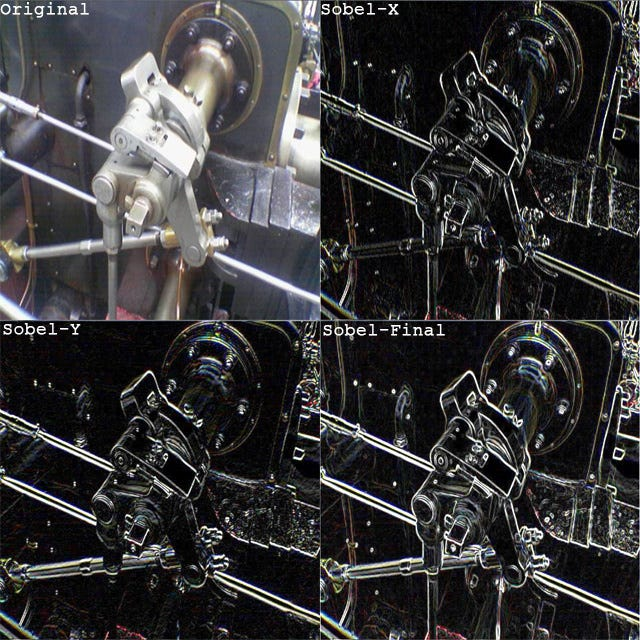From: Wikipedia

Or we could use filters to reduce noise, by taking the median, which would be something like this: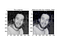From: StackOverflow

Which works by sorting the values in a 3x3 cut of the image and taking the middle value, like this: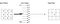From: FPGA Implementation of Median Filter

Or even a bilateral filter which can really denoise a signal or image or smooth it out, like this: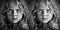From: Bilateral Noise Reduction

Where you can see it preserves the edges well but smooths it.

So if we look at the vertical edge filter, for example, we can see that same filter can be applied to the whole image, so a simple 3x3 filter can be used in the whole image to detect the vertical edges.

## Choosing the filters

If the paradigm of the problem is well known maybe we can hand engineer some filters to process the data as we need. Sometimes this is hard, what if we could get the computer to learn the necessary filters for the desired task, like looking at a X-Ray picture and detecting some disease or not (assuming no previous knowledge about the disease). Enter CNNs! By using CNNs we will get the computer to learn the necessary filters he needs to do the detection by himself.

# Convolutional layer

So that is what convolutional layers do, they figure out filters that will “stack” in a network to discover complex information. They capture Spatial and Temporal dependencies in an image by applying the filters.

## Padding

What about the corner of the images? Those only appear once in the convolution, how can we make them appear more and have more weight for other points as well? We can pad the image!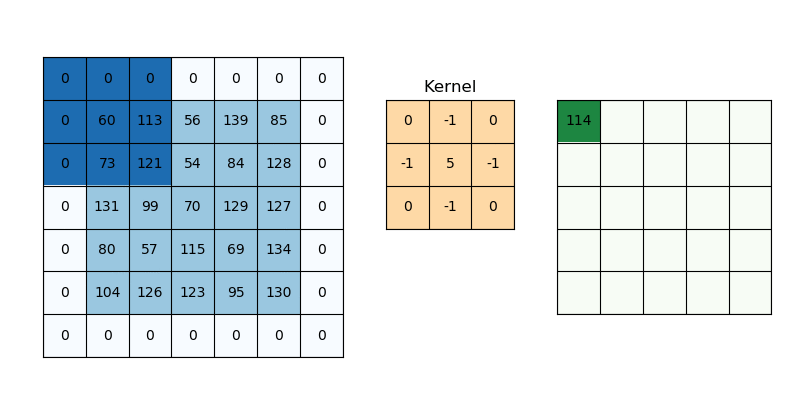From: Keras Conv2D

This solves this issue!

## Stride

We can apply the convolution to each pixel, going one by one or we could take bigger steps, known as stride. Let’s say we take a stride of 2, so we jump 2 pixels every time: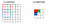From: A Beginner’s Guide To Understanding Convolutional Neural Networks Part 2

## Let’s talk sizes

So what is the size of the outputs?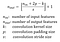From: Medium

The “brackets” around the expression mean floor, to round down.

So let’s check the previous example: 7x7 image, stride=2, kernel_size=3 and padding=0.

This gives us: floor((7–3)/2) + 1 = 2 + 1 = 3.

So we get a 3x3 output, as expected. So we can really use stride to reduce our output size if we want/need to.

## Taking conv over volume

So far we have seen the case of nxnx1 images, what if our images are nxnx3 (RGB images), how does it work then?

So now our filters instead of 3x3 will have to be 3x3x3 and it will be like this:

So we have filter 1 and we apply it to each of the 3 channels of our input image (the filter is 3x3x3 because it’s the same filter which is 3x3, but 3 times, one for each channel of the input), we then sum it up which will generate the 4x4 matrix we have, remember the output size will be floor((6–3)/1) + 1 = 4. So it will be 4x4 but since we have 2 filters our output will be 4x4x2, 4x4 for each filter and then the number of filters.

This is usually represented as 3d Shapes, like this: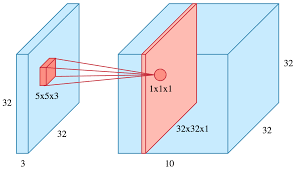From: KDNuggets

In this example we have a 5x5 filter, and we have 10 of them. In this case the output size is the same as the input size. This is a filtering called “same” filtering, because it keeps the same dimensions as the input, this is achieved by the use of padding. The filters you saw before where the size changes are called “valid” filters.

# Pooling layers

Conv layers can get big, having unnecessary information and get computationally costly. How can we get dominant features from the Conv layer and reduce its size? By applying Pooling!

There are two types of pooling, max and average. Let’s look at Max Pooling first

## Max Pooling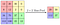From: Papers With Code

We have our 4x4 image and we will use a Pooling layer with size 2, so 2x2 filter. This will take regions of 4 numbers and take the max value of those numbers, by doing so we reduce the size of the input in half! So our 4x4 image becomes a 2x2 image!

## Average Pooling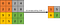From: Average Pooling

For average pooling we have the 2x2 filter, we take regions of 4 pixels and average them out. In pooling the stride is usually the size of the filter, but you can change it and get different output sizes, like before.

Choosing the pooling layer

Max Pooling is pretty much dominant these days since its a lot cheaper to compute with same or better performance than average pooling.

# Flatten Layers

This is the last type of layers in our CNN and they basically do what their name implies, they flatten the data, like so: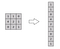From: Super Data Science

# Bulding a Conv Net

Now all we have to do is take these layers and build our own network!

We take some Conv and pool layers, we flatten them and then we use Fully connected layers, as we do in MLP.

Example of a CNN: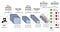From: Towards Data Science

You can, and its customary, to use ReLu activations, or others, to introduce non-linearity either after the Conv or the Pool layer.

The regular structure of a conv net is Conv->Pooling->ReLu->Conv->Pooling->Relu….->Flatten->Fully connected->Fully connected.

# Why should you care about it?

So why should you use a CNN instead of a MLP? You could just take the image, flatten it and put it through fully connected layers, so why not?

The number of parameters in a MLP can really grow fast, say we have a 32x32x3 image and we use 6 5x5 filters, generating a 28x28x6 output. 32x32x3=3072 and 28x28x6=4074. So if we were going to create a MLP with 3072 nodes in one layer and 4074 nodes in another layer and connect them all, the weight matrix would be 3072x4704 which is around 14M. That’s a lot of parameters and that’s only two layers, if the images were bigger this would get really infeasible. If we look at the parameters in a conv layer each filter is 5x5 so it has (5x5x3) parameters plus a bias, so 76 parameters, since we have 6 filters, the parameters are 456. So the number of parameters really is small, this is because, like I mentioned before, the filters take into account that, what works for a part of the image probably also works for another part, so there would be a lot of redundant parameters in an MLP. Another good reason why CNNs converge faster and work so well is that in each layer the output values depend on a small number of inputs. MLP also disregard spatial information since it takes flattened vectors as the inputs.

# How to use them

CNNs are usually very data hungry, so we need tools to augment the data we have if we can’t get more data.

For instance let’s take a look at this dataset from Kaggle. In this dataset we have X-Ray pictures of the chest area of people with and without pneumonia and, the goal is to create a classifier that can detect this.

Which is composed of images like the previous one. So before we feed this images into our model it’s probably a good idea to maybe zoom in some areas, shift the image, maybe rotate it a bit so the network can really understand the queues it needs to detect it. In order to do this we can use ImageDataGenerator from tensorflow, like this:

We could then take a network like the following:

I also did a diagram so you can visualize the network I implemented (broke it into two lines for easier visualization):

This network got 87% on test and 93% on train. A bit of overfit, most networks there were also in this neighborhood of 85%-88%.

# Transfer Learning

A method that is very common in the field of Computer Vision is transfer learning. Say you have a small amount of data to train for a task, a good idea might be to simply use someone else’s network, remove the fully connected layers and add your own. If you have a bit more data you can also train some layers in the network you imported, or all of it. I also used transfer learning for the previous dataset and got a similar result.

I used the VGG19 network. You can import it in tensorflow like this:

You can take networks from papers like AlexNet, LeNet, Inception, ResNets or more. I will not go into further detail in this topic so the post doesn’t get too long but it’s important that you know of this method.

# Next Steps

If you’d like to know more about CNN and the field of computer vision I would recommend you do the course Convolutional Neural Networks.

Originally published at https://joao-maria-janeiro.github.io on Jan 27, 2021.

2x Software Engineering intern @ Google | Electrical and Computer Engineering Student at Instituto superior Técnico

## More from João Maria Janeiro

2x Software Engineering intern @ Google | Electrical and Computer Engineering Student at Instituto superior Técnico